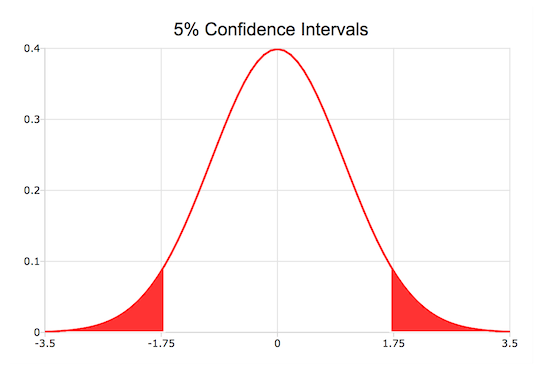## Purpose¶

Adds a cumulative area plot to an existing graph.

## Format¶

plotAddArea([myPlot, ]x, y[, base])
Parameters
• myPlot (struct) – Optional argument, a plotControl structure.

• x (Nx1 vector) – the X coordinates

• y (Nx1 or NxM matrix) – Each column contains the height for the corresponding section of the graph. If y contains more than one column, each column will be stacked on top of the previous column.

• base (scalar) – Optional argument. The height for the base of the added area plot. The default value is zero. plotAddArea() does not yet support a vector input for base.

## Examples¶

### Creating confidence intervals¶

// Create the 'x' and 'y' for the normal
// probability density function
x = seqa(-3.5, 0.1, 71);
y = pdfn(x);

// Create the 'x' and 'y' points
// for the left tail
edge = cdfni(0.05);
x_ci = selif(x, (x .< edge));
y_ci = y[1:rows(x_ci)];

// Set colors
struct plotControl myPlot;
myPlot = plotGetDefaults("xy");

clr = getColorPalette("accent", 3);

plotSetLineColor(&myPlot, clr);

plotSetFill(&myPlot, 1, 1, clr);

// Draw filled in left tail
plotArea(myPlot, x_ci, y_ci);

// Create the 'x' and 'y' points
// for the right tail
edge = cdfni(0.95);
x_ci = selif(x, (x .> edge));
y_ci = y[rows(y)-rows(x_ci)+1:rows(y)];

// Add right tail to graph

plotSetLineSymbol(&myPlot, -1);

Creating confidence intervals with plotAddArea()plotAddArea() may only add curves to 2-D graphs.